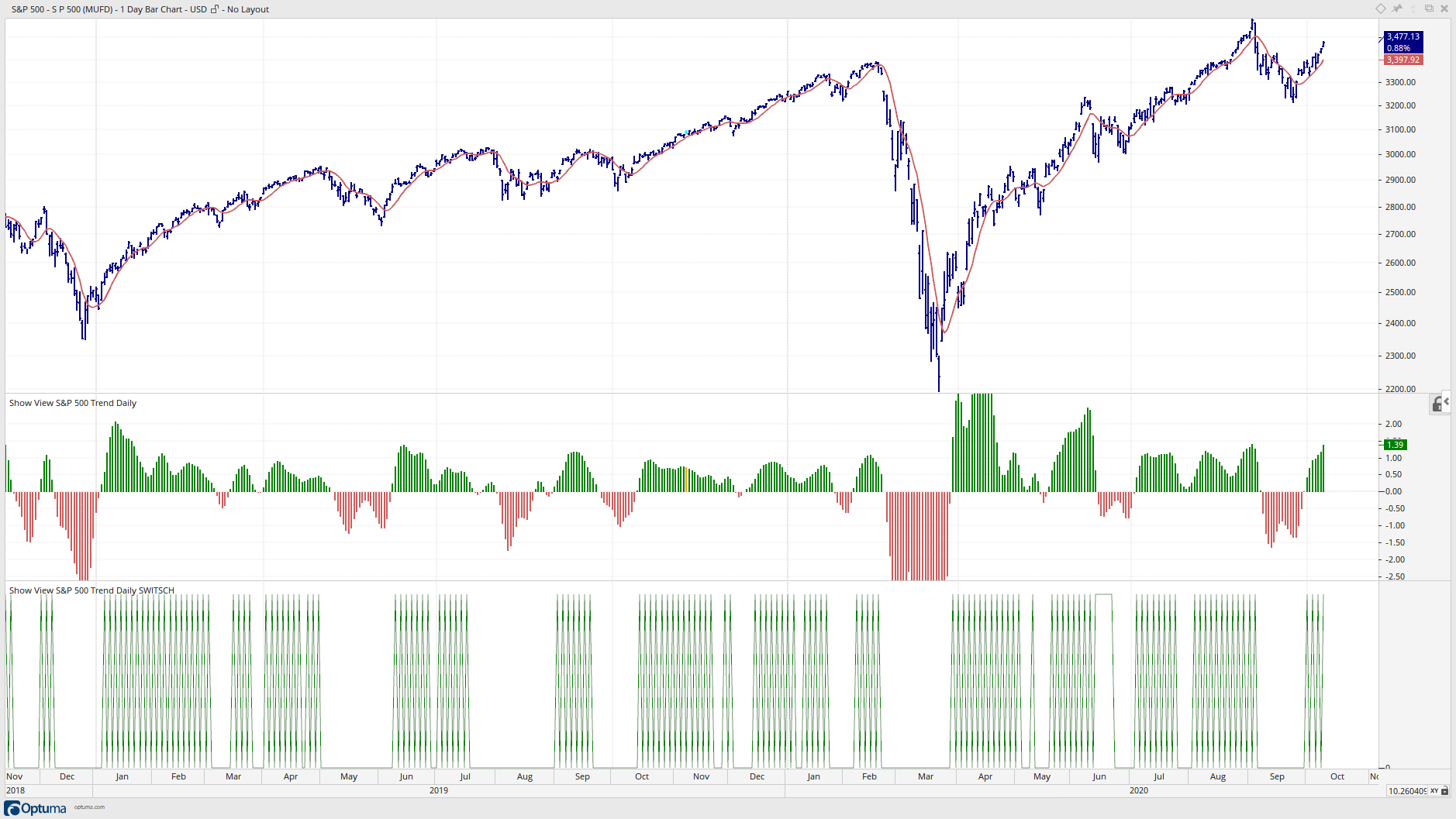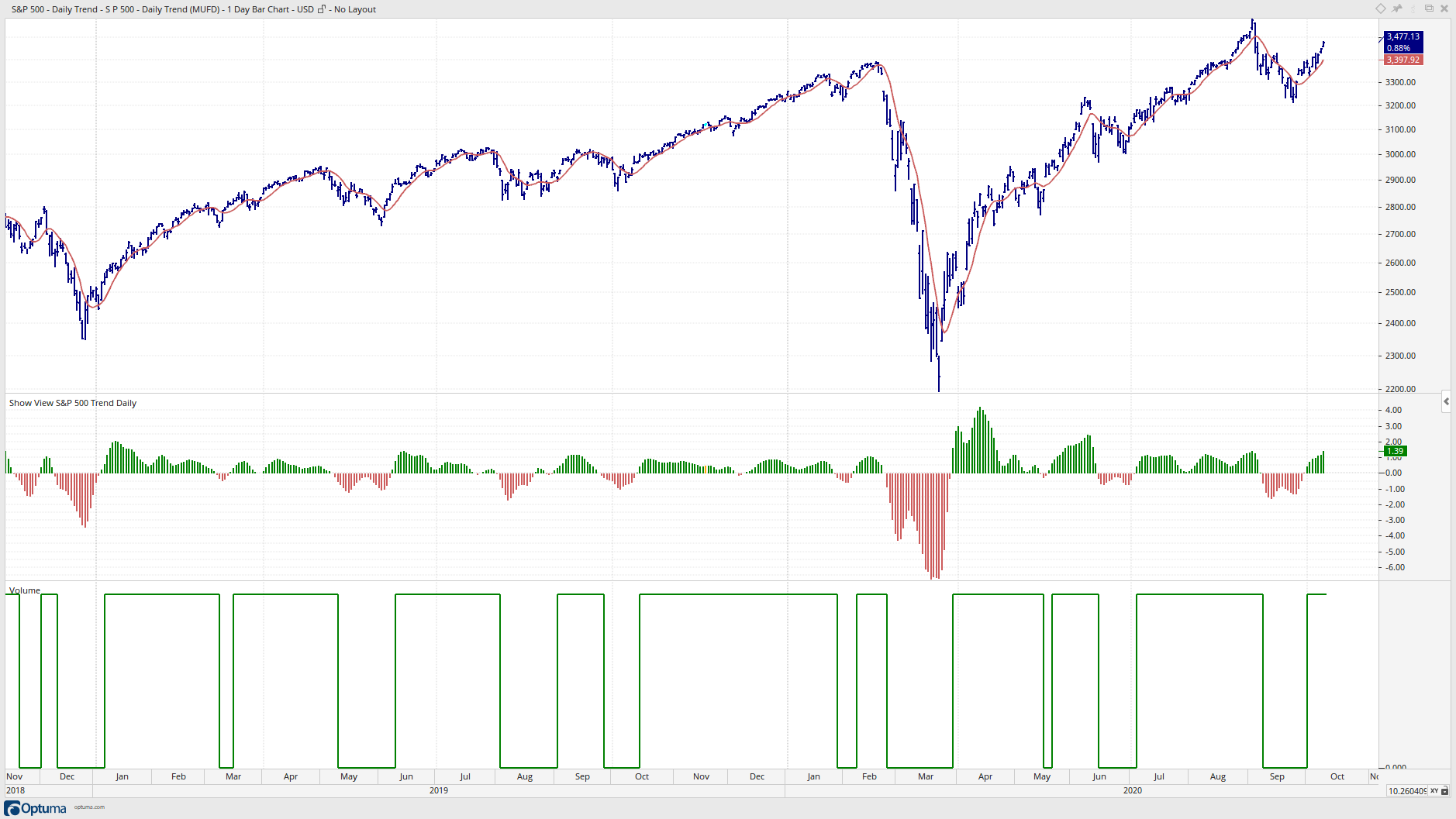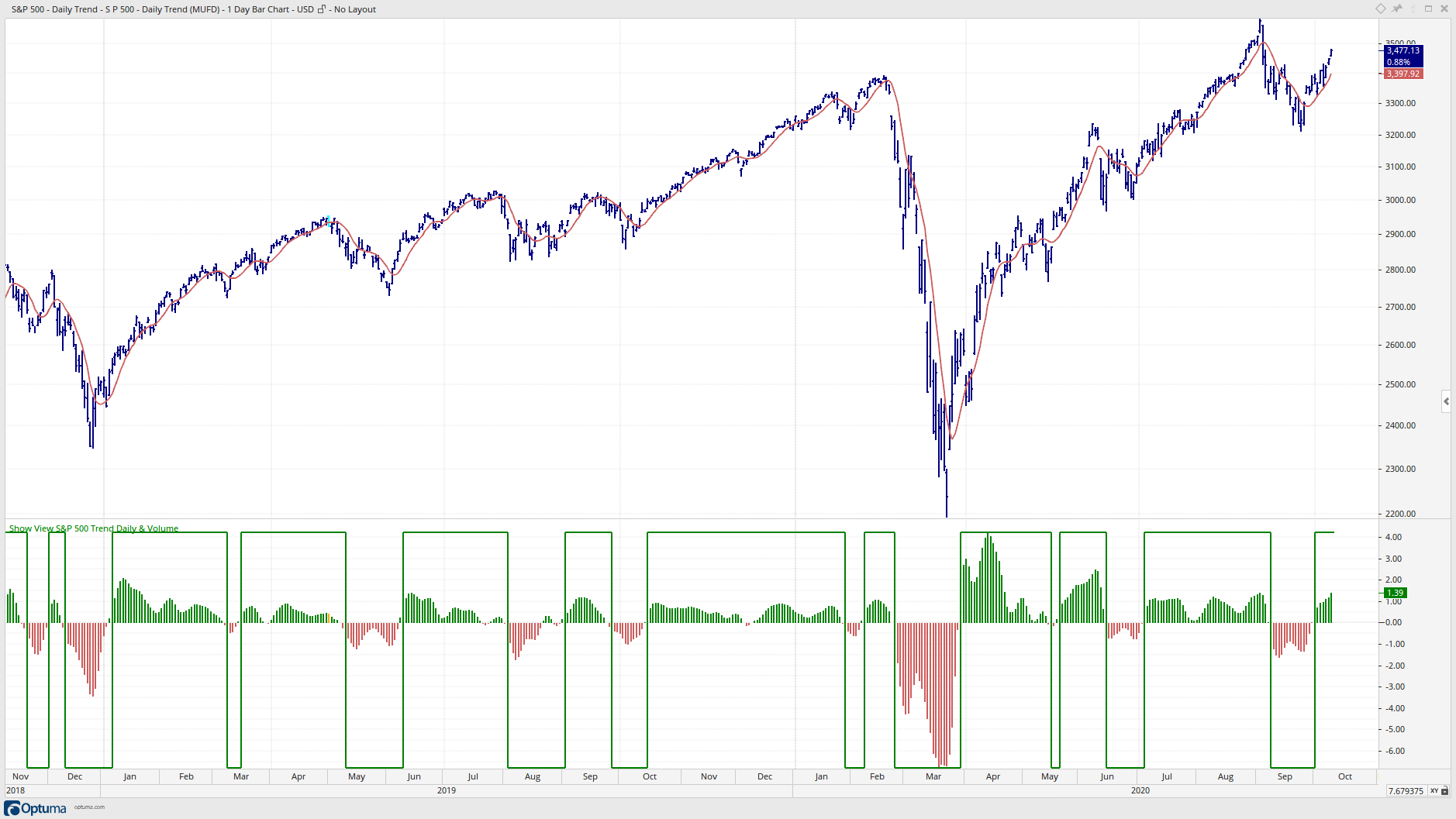Select Page

Optuma Forums Optuma Scripting IF Statement Reply To: IF Statement

#60315

Hi Mathew,

thanks for your replay. Using your Var4 recommendation I have to say that it does not what it should.

Here is the script with your recommendation:

This the the chart with the above script.The histogram is the following script:

I have done the job in Excel, the Excel file is attached. Here are the charts how it should be.I have saved the trend values “1” and “0” in the volume column of the csv file and then have displayed the “volume” in Optuma. The volume is the trend indicator. The csv file is also attached.

Here are the description what the trend indicator should do and how it is calculated:
Var2: I calculate a 8 period Exponential Regularization Moving Average
Var3: Percent change of the Exponential Regularization Moving Average over the last three days
Var4: The trend indicator should have a value of “1” as long as Var3 is above minus 0.3 and when the Var3 is negative more than minus 0.3 the trend indicator should have the value “0” as long as Var3 is below 0.3.
When Var3 moves the first time above 0.3 and the trend indicator value was the bar before “-1” it turns to “1”.
When Var3 moves the first time below minus 0.3 and the trend indicator value was the bar before “1” it turns to “-1”.

The problem is the range between 0.3 and minus 0.3. This is the range where often whipsaws occur and to remove these whipsaws makes the issue a little bit complicated.

I hope I have clarified the issue, if not please let me know.

Thanks
Thomas